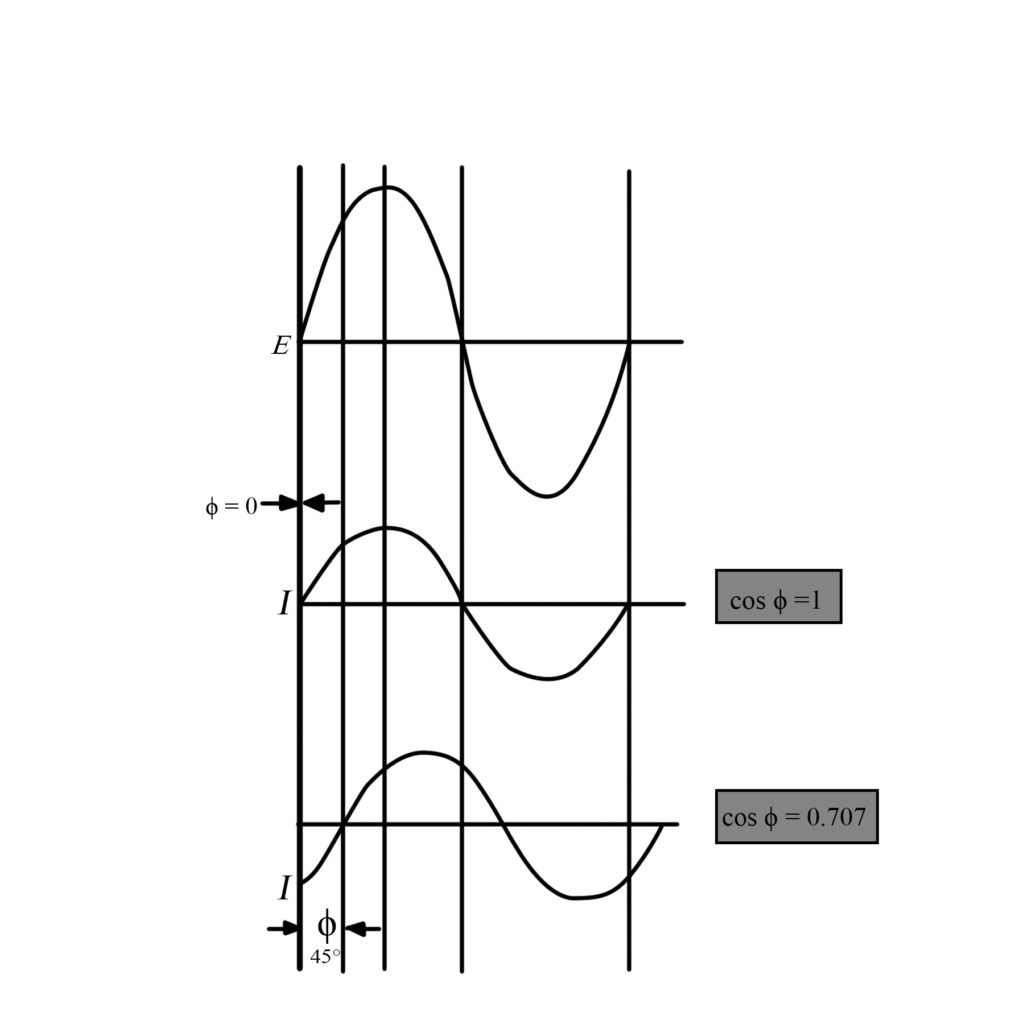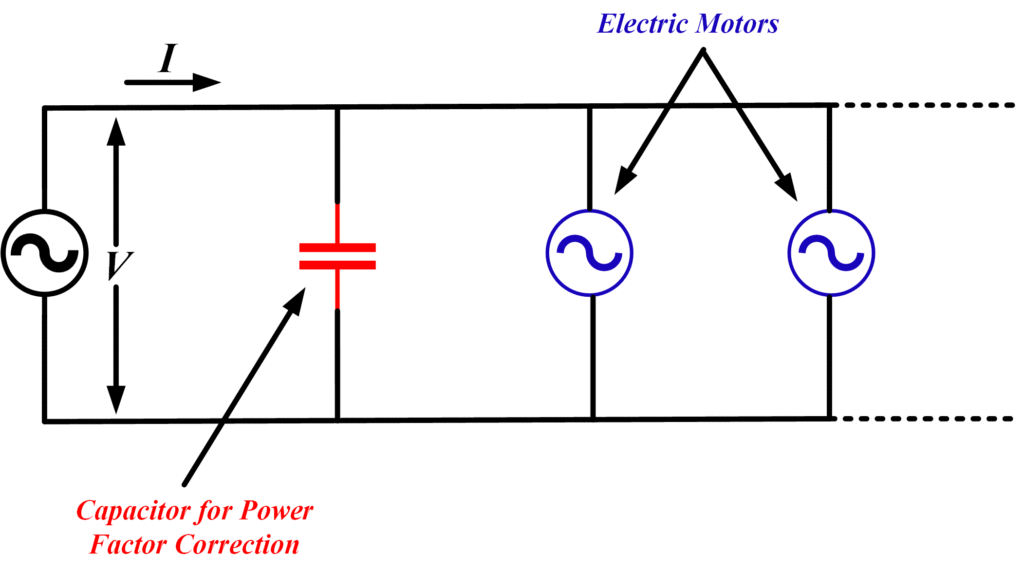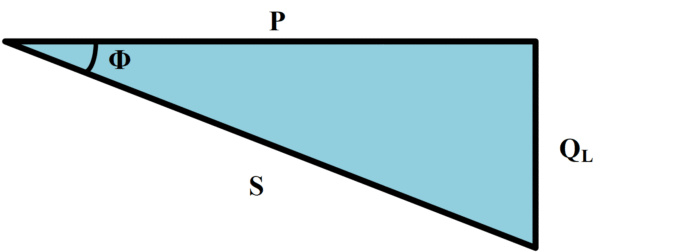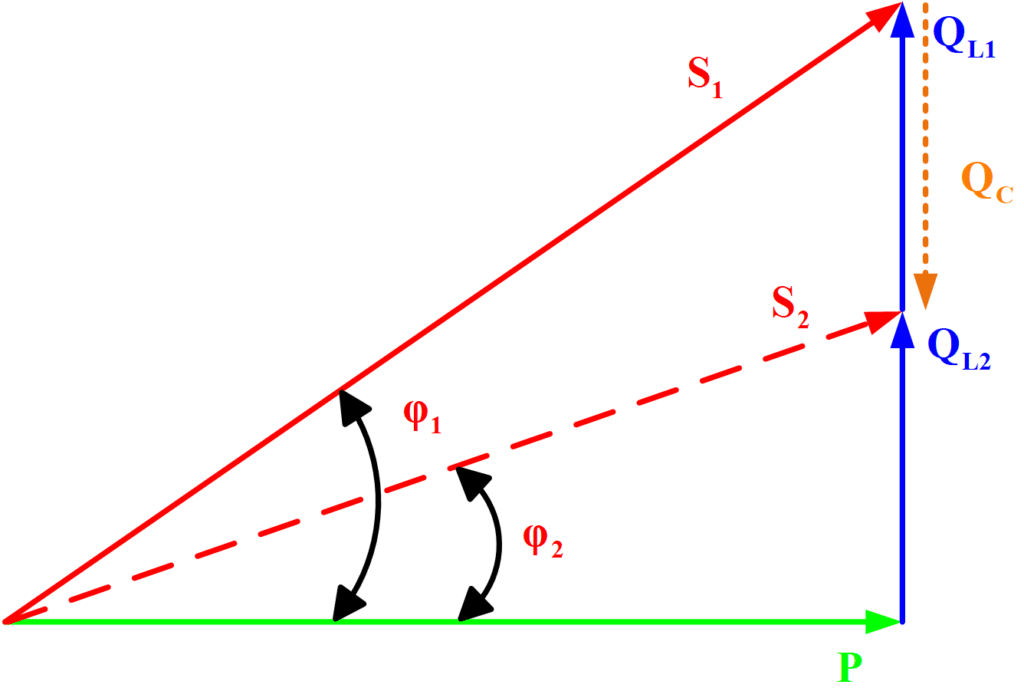# Power Factor Correction using Capacitor Bank

Want create site? Find Free WordPress Themes and plugins.

## Power factor

Ideally, all the supply voltage and current should be converted into true power in a load. When this is not a case, a certain kind of inefficiency occurs. The ratio of true power to apparent power is called the power factor of the load,

$\begin{matrix} Power\text{ }Factor=\frac{true\text{ }Power}{Apparent\text{ }Power}=\frac{P}{EI} & {} & (1) \\\end{matrix}$

The true power in a load is calculated from the following equation,

$\begin{matrix} P=EI\cos \phi & {} & (2) \\\end{matrix}$

Where E and I are supply voltage and current and ϕ is the phase angle between them.  Equation (2) can be rewritten to give:

$\cos \phi =\frac{P}{EI}$

Therefore,

$\begin{matrix} Power\text{ }Factor=\cos \phi =\frac{P}{EI} & {} & (3) \\\end{matrix}$

If the power factor is 1, the phase angle ϕ is zero, and all of (E×I) from the supply is dissipated as true power in the load.

Where ϕ is greater than zero, cos ϕ is less than 1, and only a portion of supply (E×I) is converted into useful power.

Thus, it is seen that the power factor has always a maximum value of 1 and is normally less than 1 (see figure 1).Fig.1: The power factor of a load is the cosine of the angle between the supply voltage and the load current.

The power factor can be expressed as a ratio or as a percentage and is also usually defined as leading or lagging. A 60 % lagging power factor implies an inductive load in which the supply current lags the voltage by an angle with a cosine of 0.6 (i.e., approximately 53o).

A 90 % leading power factor would indicate a capacitive load in which the current leads the voltage by an angle with a cosine of 0.9, or approximately 26o.

## Power Factor Correction

Most industrial loads consist of electric motors. Thus, they are inductive and have lagging power factors. Consider the situation illustrated in figure 2, and assume that the supply voltage and current measured as E=120 V and I=100 A, with the current lagging the voltage by an angle of 33.5o. Using the appropriate equations, the apparent power, true power, and reactive power are calculated as:Fig.2: generator with an inductive load

Apparent power,

$S=EI=120\times 100=12kVA$

True power,

$P=EI\cos \phi =12kVA\times \cos ({{33.5}^{o}})=10kW$

Inductive reactive power,

$Q=EI\sin \phi =12kVA\times \sin ({{33.5}^{o}})=6.6kVAR$

A power triangle can be drawn for the circuit, as illustrated in figure 3.Fig.3: power triangle for an inductive load

The true power P is represented by a horizontal vector, and the vector for an apparent power S is drawn lagging the true power by the phase angle ϕ. The reactive power vector QL completes the triangle.

Because electric motors are inductive, the supply current lags the supply voltage; they are said to have a lagging power factor. A power triangle can be drawn to show the relationship between true power P, apparent power S, and reactive power QL.

If the power factor were 1 (i.e., ϕ=0), then to supply a power of 10 kW to the load would require a current of:

$I=\frac{P}{E}=\frac{10kW}{115V}=83A$

This shows that if the power factor could be increased to unity (ϕ reduced to zero), the generators supplying the load would have to produce the current of only 83 A instead of 100 A while still supplying the required amount of true power.

Also, the conducting cables could be selected to carry 83 A instead of 100 A, and consequently, they would be less expensive. Thus, it is always best to have a power factor as near unity as possible, and in fact, the power factor of an inductive load can be adjusted toward unity. This process is known as power factor correction.

Power factor correction for an inductive load consists simply of connecting capacitance in parallel with the load. Suppose that the 100 A load had been capacitive instead of inductive. The current would then lead the voltage by the phase angle, and the power triangle would be drawn as illustrated in figure 4.Fig.4: power triangle for a capacitive load

The apparent power vector S is shown leading the true power vector P by the angle ϕ, and the capacitive reactive power QC is drawn vertically up from the true power vector, as shown.

When the capacitance is connected in parallel with an inductive load, the power triangle has a capacitive reactive power component as well as well as inductive reactive power. The diagram in figure 5 illustrates the situation. In reference to the power triangle, the parallel capacitor supplies a reactive power, QC, which cancels some of the original reactive power, QL1, leaving a net inductive power QL2 .Accordingly, the apparent power is decreased from S1 to S2. Notice that if an overall power factor of unity is desired, the capacitor is chosen so that its reactive power equals the reactive power of the inductive load. In such a case;

$P=S~~~~~~~~~~~~~~~~~~~~~~~~~~~~~~~~~~~at\text{ }Cos\theta =1$Fig.5: Power Factor Correction using Capacitor

## Example of Power Factor Correction Calculation

A load operating at a lagging power factor of 0.7 dissipates 2 KW when connected to a 220 V, 60 Hz power line. What value of capacitance is needed to correct the power factor to 0.9?

Solution

Referring to the given data and above mentioned figure,

${{\theta }_{1}}=Co{{s}^{-1}}\left( 0.7 \right)={{45.6}^{{}^\circ }}$

${{\theta }_{2}}=Co{{s}^{-1}}\left( 0.9 \right)={{26}^{{}^\circ }}$

Then,

${{Q}_{1}}=Ptan{{\theta }_{1}}=2000*tan({{45.6}^{{}^\circ }})=2040\text{ }~vars$

${{Q}_{2}}=Ptan{{\theta }_{2}}=2000*tan({{26}^{{}^\circ }})=975~\text{ }vars$

And

${{Q}_{C}}={{Q}_{1}}-{{Q}_{2}}=1065~\text{ }vars$

From$~~~~{{Q}_{C}}=\frac{{{V}^{2}}}{{{X}_{c}}}$ ,

${{X}_{C}}=\frac{{{V}^{2}}}{{{Q}_{C}}}=\frac{{{200}^{2}}}{1065}=\frac{4.84*{{10}^{4}}}{1.065*{{10}^{3}}}=45.4~\Omega$

And

$C=\frac{1}{\omega {{X}_{C}}}=\frac{1}{2*\pi *f*45.4}=58.3~\mu F$

In view of the increased current that must be supplied when the power factor is less than 1, electric utilities often charge higher rates to large industrial consumers whose plats operate at low power factors.

The sign of Q depends upon the nature of the load’s reactance. If the load is inductive, so that Q>0 and ϕ>0, then we say that it has a lagging power factor, meaning that the load current lags the supply voltage. Conversely, if the load is capacitive, so that Q<0 and ϕ<0, then we say that it has a leading power factor, meaning that the load current leads the supply voltage.

Most industrial loads include inductive components, such as motor windings, and therefore have lagging power factors. Capacitors are often used in conjunction with those loads for the purpose of power factor correction. The capacitor is connected in parallel with the loaf to avoid an unwanted voltage drop. But an appropriate capacitor in parallel with an inductive load cancels out the reactive power, and the combined load has a power factor equal to 1, thereby minimizing current drawn from the source.

Did you find apk for android? You can find new Free Android Games and apps.